## 1. Introduction

We often need to be able to match two different sets of data together, such that as many elements as possible from one set are associated with an element from the other set.

For example, imagine scheduling appointments into available slots. If a professor has a fixed number of time slots available for consultations, and a number of students who want to take advantage of them, then we need to work out the most efficient way to match these together.

## 2. Maximum Bipartite Matching

A bipartite graph is a special case in graph theory because every vertex is in one of two distinct sets, often calledand, and every edge connects betweenand. We can consider our two sets of data that we’re matching up to be the two setsand. The edges between the two are then the allowed connections.

For example, in our case of a professor’s time slots and students, the vertices incould be the individual students, the vertices incould be the available slots, and the edges indicate which time slots each student is able to use: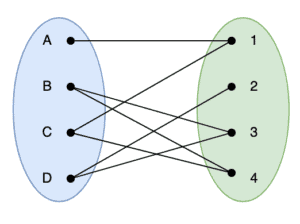A matching in graph theory, also known as an independent edge set, is a combination of edges where no two edges share the same vertex. This will often be a subset of all possible edges to then meet this requirement. The “maximum matching” is a matching that has the most number of edges possible. A “perfect matching” is one that uses every vertex in the graph, and therefore is the largest possible matching that we can achieve.

As such, the maximum matching of a bipartite graph is the maximum number of possible links between the two distinct sets, so that every link is between a different set of vertices. This can be a very useful concept since this is effectively the maximum number of pairings between two related sets; like between students and appointment slots for example.

## 3. Hopcroft-Karp Algorithm

The Hopcroft-Karp algorithm is an algorithm to generate a maximum matching for a given bipartite graph. This was developed by John Hopcroft and Richard Karp in 1973.

The algorithm works in phases, where each phase is looking for augmenting paths of increasing lengths. An augmenting path is any path starting and ending on unmatched vertices, where edges within the path alternate between unmatched and matched. We will always have an odd number of edges in these paths, but they could be of size 1 or up.

Each path we find is then used to increase the matching that we have so far. We do this by adding all previously unmatched edges into our matching, whilst removing all previously matched edges from the matching. This will have the effect of increasing the size of the matching by 1 for every path found.

For example, if we started from the following: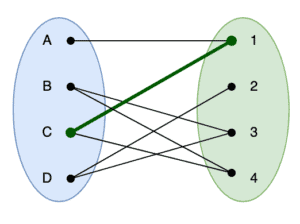Here we have a single path between verticesand. We can find a new path that travels from, and add this to our matching. Doing so will end up with the following: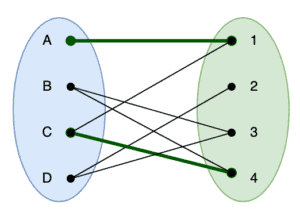Here we can see thatgets removed from the matching, whilst addingandinto it. The end result is that we now have 2 paths in the matching.

## 4. Algorithm

The algorithm works in phases, where we perform a number of searches across the graph in each phase. We also do everything from the point of view of the vertices in, which helps simplify the number of steps required.

As input, the only thing that we require is the graph itself. Specifically, the details of which vertices inconnect to which vertices in. Throughout the entire algorithm, we will also keep track of the matching. Specifically, which vertices inare currently paired with vertices in, though we need to be able to access this from both sides.

For each phase, we will additionally be keeping track of the current paths we have discovered. This is recorded as distances along the path for each vertex in. We don’t need to record distances for vertices inbecause these are only ever considered from the point of view of how they connect to vertices in.

### 4.1. Overall Algorithm

The overall algorithm starts with some general set up and then performs a number of searches grouped together in phases. During each phase, we perform a breadth-first search of the entire graph to discover any augmenting paths that exist in the graph. We then perform a number of depth-first searches, starting from each vertex inthat is currently unmatched to build the appropriate augmenting paths and update the matching.

We also have a special vertex that is not part of the graph but is used in the algorithm. This is referred to as NIL, and we use this to indicate that a vertex in the graph is not currently paired with anything:At the start of each phase, we perform a breadth-first search across the entire graph to discover if any augmenting paths exist, and the lengths of them. If we did not calculate a distance to the special NIL vertex, which indicates that we didn’t find any paths, then we will stop immediately. This means we have already reached the best matching possible.

If we did find an augmenting path, then we will expand our matching. This is done by iterating over every vertex inthat is not currently paired and running a depth-first search across the graph starting from there. As part of this, we will be updating the matching, and swapping edges in and out as appropriate.

The first part of each phase is the breadth-first search. We iterate over every vertex inand expand out to discover all of the augmenting paths that we can:We first discover every vertex inthat is not currently part of the matching and add them to a working queue. We then iterate over this queue, looking at each vertex in turn.

For each of these, we iterate over every attached vertex infrom the graph. We find the vertex fromthat is currently paired to this, which may be our special NIL vertex. If we’ve not yet calculated a distance for this, then we do so and add it to the working queue.

This then repeats until we run out of vertices to examine. At this point, we know every possible augmenting path through the graph from our current state, and we’re ready for the next step.

Once we’ve generated the current set of augmenting paths, we will start to expand them. We do this by performing a depth first search through the graph, from each vertex inthat is not currently part of the matching. As we do this, we update the matching accordingly:## 5. Working Example

This is complicated, so the best way to follow it is to actually work along. Here we are using a very simple graph to make the demonstration shorter, but it will expand out to graphs of any size: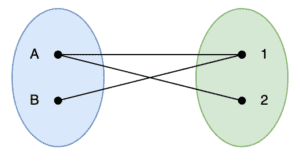Our graph has two vertices on each side, and three edges between them. For example, it could represent a professor with morning and afternoon appointments available, and two students wishing to make use of them.

### 5.1. Phase 1

When we do the very first breadth-first search, this will discover a single augmenting path through the graph. This is between verticesand. We don’t find any paths using verticesor, because the only possible paths are using vertices that have already been used.

After this, we then perform depth-first searches starting from bothand. The search starting fromwill add the edgeinto the matching, and the search starting fromwill add nothing: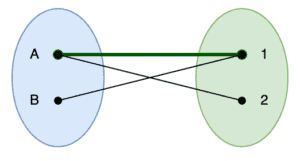### 5.2. Phase 2

Our second breadth-first search will be starting from the above graph instead. In this case, we don’t need to investigate any paths frombecause it’s already in the matching, and so we instead start from. This will now discover the augmenting path that travels from.

After this, we again perform the depth-first searches. As before, we only do this fromsinceis already in the matching, and this will then addandinto the matching, whilst at the same time removingfrom the matching: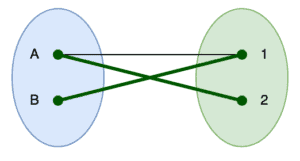### 5.3. Phase 3

Our third breadth-first search has no vertices to start from because bothandare already included. This means that we stop processing and return the matching that we have discovered so far. We can already see from looking at the above that this is the optimal matching.

## 6. Summary

In this article, we learned how we can match up two sets of data such that the most effective matching between them can be discovered. We’ve demonstrated it working on a small scale, but it will work equally effectively on much larger graphs as well.